So Gainwell has let me open source one of the projects I have been working on at work – a python package to download SVI data. The SVI is an index created by the CDC to identify areas of high health risk in four domains based on census data (from the American Community Survey).For my criminologist friends, these are very similar variables we typically use to measure social disorganization (see Wheeler et al., 2018 for one example criminology use case). It is a simple python install, `pip install svi-data`. And then you can get to work. Here is a simple example downloading zip code data for the entire US.

``````import numpy as np
import pandas as pd
import svi_data

key = svi_data.get_key('census_api.txt')

# Download the data from census API
svi_zips = svi_data.get_svi(key,'zip',2019)
svi_zips['zipcode'] = svi_zips['GEO_ID'].str[-5:]``````

Note I deviate from the CDC definition in a few ways. One is that when I create the themes, instead of using percentile rankings, I z-score the variables instead. It will likely result in very similar correlations, but this is somewhat more generalizable across different samples. (I also change the denominator for single parent heads of households to number of families instead of number of households, I think that is likely just an error on CDC’s part.)

## Summed Index vs PCA

So here quick, lets check out my z-score approach versus a factor analytic approach via PCA. Here I just focus on the poverty theme:

``````pov_vars = ['EP_POV','EP_UNEMP','EP_PCI','EP_NOHSDP','RPL_THEME1']
svi_pov = svi_zips[['zipcode'] + pov_vars ].copy()

from sklearn import decomposition
from sklearn.preprocessing import scale

svi_pov.corr()``````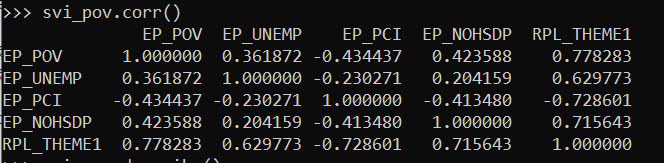Note the per capita income has a negative correlation, but you can see the index works as expected – lower correlations for each individual item, but fairly high correlation with the summed index.

Lets see what the index would look like if we used PCA instead:

``````pca = decomposition.PCA()
sd = scale(svi_pov[pov_vars[:-1]])
pc = pca.fit_transform(sd)
svi_pov['PC1'] = pc[:,0]
svi_pov.corr() #almost perfect correlation``````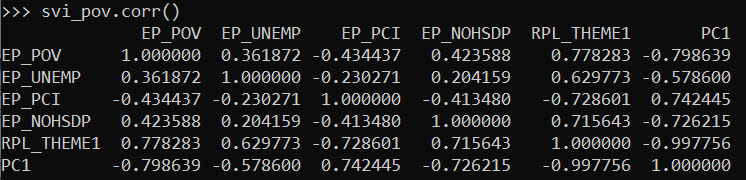You can see that besides the negative value, we have an almost perfect correlation between the first principal component vs the simpler sum score.

One benefit of PCA though is a bit more of a structured approach to understand the resulting indices. So we can see via the Eigen values that the first PC only explains about 50% of the variance.

``print(pca.explained_variance_ratio_)``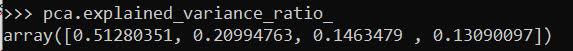And if we look at the loadings, we can see a more complicated pattern of residual loadings for each sucessive factor.

``````comps = pca.components_.T
cols = ['PC' + str(i+1) for i in range(comps.shape)]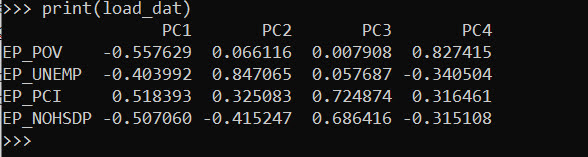So for PC3 for example, it has areas with high no highschool, as well as high per capita income. So higher level components can potentially identify more weird scenarios, which healthcare providers probably don’t care about so much by is a useful thing to know for exploratory data analysis.

## Mapping

Since these are via census geographies, we can of course map them. (Here I grab zipcodes, but the code can download counties or census tracts as well.)

We can download the census geo data directly into geopandas dataframe. Here I download the zip code tabulation areas, grab the outline of Raleigh, and then only plot zips that intersect with Raleigh.

``````import geopandas as gpd
import matplotlib.pyplot as plt

# Getting the spatial zipcode tabulation areas
zip_url = r'https://www2.census.gov/geo/tiger/TIGER2019/ZCTA5/tl_2019_us_zcta510.zip'
zip_geo.rename(columns={'GEOID10':'zipcode'},inplace=True)

# Merging in the SVI data
zg = zip_geo.merge(svi_pov,on='zipcode')

# Getting outline for Raleigh
ncp_url = r'https://www2.census.gov/geo/tiger/TIGER2019/PLACE/tl_2019_37_place.zip'
ral = ncp_geo[ncp_geo['NAME'] == 'Raleigh'].copy()
ral_proj = 'EPSG:2278'
ral_bord = ral.to_crs(ral_proj)

ral_zips = gpd.sjoin(zg,ral,how='left')
ral_zips = ral_zips[~ral_zips['index_right'].isna()].copy()
ral_zipprof = ral_zips.to_crs(ral_proj)

# Making a nice geopandas static map, zoomed into Raleigh

fig, ax = plt.subplots(figsize=(6,6), dpi=100)

# Raleighs boundary is crazy
#ral_bord.boundary.plot(color='k', linewidth=1, edgecolor='k', ax=ax, label='Raleigh')
ral_zipprof.plot(column='RPL_THEME1', cmap='PRGn',
legend=True,
edgecolor='grey',
ax=ax)

# via https://stackoverflow.com/a/42214156/604456
ral_zipprof.apply(lambda x: ax.annotate(text=x['zipcode'], xy=x.geometry.centroid.coords, ha='center'), axis=1)

ax.get_xaxis().set_ticks([])
ax.get_yaxis().set_ticks([])

plt.show()``````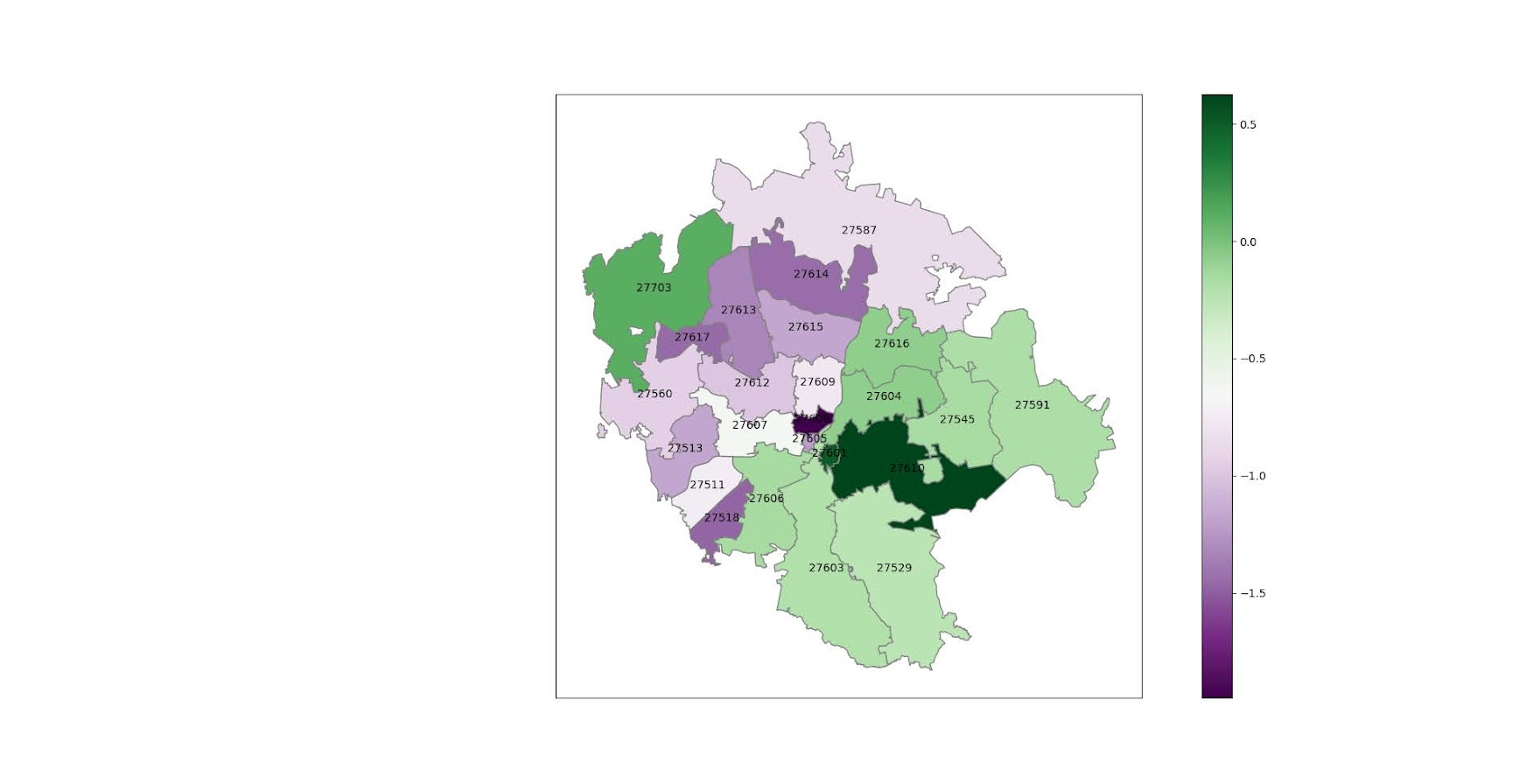I prefer to use smaller geographies when possible, so I think zipcodes are about the largest areas that are reasonable to use this for (although I do have the ability to download this for counties). Zipcodes since they don’t nicely overlap city boundaries can cause particular issues in data analysis as well (Grubesic, 2008).

# Other Stuff

I have a notebook in the github repo showing how to grab census tracts, as well as how to modify the exact variables you can download.

It does allow you to specify a year as well (in the notebook I show you can do the 2018 SVI for the 16/17/18/19 data at least). Offhand for these small geographies I would only expect small changes over time (see Miles et al., 2016 for an example looking at SES).

One of the things I think has more value added (and hopefully can get some time to do more on this at Gainwell), is to peg these metrics to actual health outcomes – so instead of making an index for SES, look at micro level demographics for health outcomes, and then post-stratify based on census data to get estimates across the US. But that being said, the SVI often does have reasonable correlations to actual geospatial health outcomes, see Learnihan et al. (2022) for one example that medication adherence the SVI is a better predictor than distance for pharmacy for example.